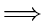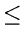Next:7.5.2 Topological SortUp:7.5 Directed Acyclic GraphsPrevious:7.5 Directed Acyclic Graphs

## 7.5.1 Test for Acyclicity

Result:

A digraph is acyclic if and only if its first search does not have back arcs.

Proof:

First we prove that

backarccycle.
Let (uw) be a backarc. This means that w is an ancestor of v. Thus (w,..., v, w) will be a cycle in the digraph.
Next we show that
cyclebackarc.
Suppose G is cyclic. Consider a cycle and let v be the vertex with the lowest dfnumber on the cycle. See Figure 7.13. Because v is on a cycle, there is a vertex u such that (uv) is an edge. Since v has the lowest dfnumber among all vertices on the cycle, umust be an descendant of v.
• it can not be a tree arc since dfnumber(vdfnumber(u)
• it can not be a forward arc for the same reason
• it can not be a cross arc since v and u are on the same cycle.
Note that the above test for acyclicity has worst case complexity O(e).Next:7.5.2 Topological SortUp:7.5 Directed Acyclic GraphsPrevious:7.5 Directed Acyclic Graphs
eEL,CSA_Dept,IISc,Bangalore